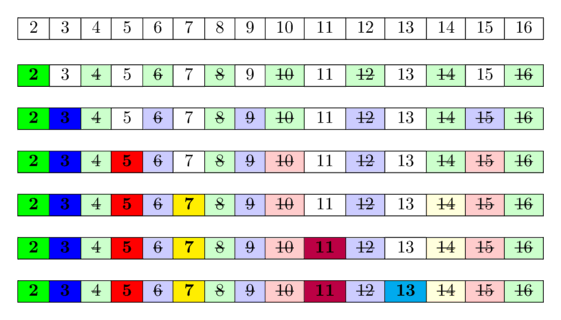## Prime number#

A prime number is a natural number with exactly 2 distinct divisors. For example 2, 3, 5, 7 and so on.

Given number N, How do we determine if it is prime? One approach is to iterate through the numbers [1, N] and count how many of them divide N. Can we check it faster than this?

If prime number has only 2 divisors, that means it s divisible by only 1 and itself. Therefore, there is no number in [2, N-1] range that can divide N. Also, N is prime if it is not divisible by any number 2 to square root of N. Because if there exist a divisor greater than the square root of N, then there must be a corresponding factor X such that N/X is less than $$\sqrt N$$. However, this is not possible because we have already checked for divisors in that interval. So, we can determine whether a number is prime by checking its divisors up the $$\sqrt N$$.

## Prime numbers up to N#

How to build prime number up to N. Let’s generate all prime numbers from 1 to N. This can be done with $$N*\sqrt N$$ operations. But of course, how to do it faster?

Let’s consider list called Prime to indicate whether number is prime. That means Prime = false, Prime true.

We know that 2 is smallest prime number. Therefore, all numbers up to N that are divisilbe by 2 cannot be prime. Let’s mark these numbers as non-prime. The next number is 3, and its prime because it is not divisible by any previous primes. Then all subsequent numbers divisible by 3 are not prime and mark all numbers divisible by 3 as non-prime. The next number 4 is marked as non-prime because it is divisilbe by 2. The next number, 5, is not marked as non-prime and is prime. Continuing this process, any numbers divisible by 5 will not be prime numbers.and our time complexity will be \begin{aligned} \sum\nolimits_{p\leq N} N/p \approx n \ln \ln n \end{aligned}

this algorithm is called Sieve of Eratosthenes. Here’s the code how to write this

#include <bits/stdc++.h>
using namespace std;

int n;
bool Prime;

int main() {
cin >> n;
Prime = 1;

for(int i = 2; i*i <= n; i++) {
if(Prime[i]) continue; // i is prime number, because it's not divisible by any previous prime

// mark all numbers divisible by i as non-prime
for(int j = i+i; j <= n; j += i) {
Prime[j] = 1;
}
}

for(int i = 1; i <= n; i++) {
if(!Prime[i]) {
cout << i << " ";
}
}
cout << endl;
return 0;
}